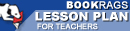Lesson Plans

# The Logic of Scientific Discovery Quiz | Eight Week Quiz D

This set of Lesson Plans consists of approximately 102 pages of tests, essay questions, lessons, and other teaching materials.
 View a FREE sampleName: _________________________ Period: ___________________

This quiz consists of 5 multiple choice and 5 short answer questions through Part II: Chapter 5, The Problem of Empirical Basis.

## Multiple Choice Questions

1. What guides a scientific experiment?
(a) Deductive reasoning.
(b) Logic.
(c) Inductive reasoning.
(d) Theory.

2. According to Popper, what can't universals be reduced to?
(a) Infinite regress.
(b) Psychologism.
(c) A class of experiences.
(d) Dogmatism.

3. What challenges Poppers use of falsification as criteria in deciding is a theoretical system is empirical?
(a) Competence.
(b) Theoretical experiments.
(c) Construction of Nature.
(d) Conventionalism.

4. What does Popper say about positivism?
(a) It is too narrow, limited, and misguided.
(b) It is a clear way of thinking.
(c) It is based on scientific data.
(d) It is based on invalid assumptions.

5. What is the theory of theories?
(a) Existential statements.
(b) System of theories.
(c) Causality.
(d) The logic of science.

1. What is the reproducible effect which refutes a theory?

2. What should the rules of epistemology adhere to?

3. What does Popper feel is a fruitless exercise?

4. What is the concept of simplicity a part of according to Popper?

5. What types of statements have no logical justification in which to draw conclusions?

 This section contains 183 words (approx. 1 page at 300 words per page) View a FREE sample